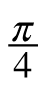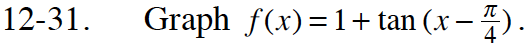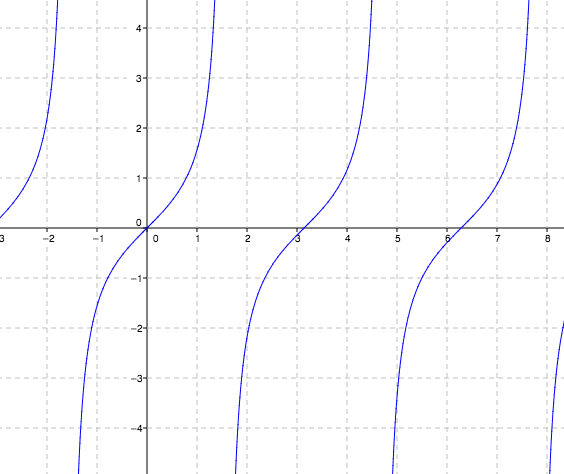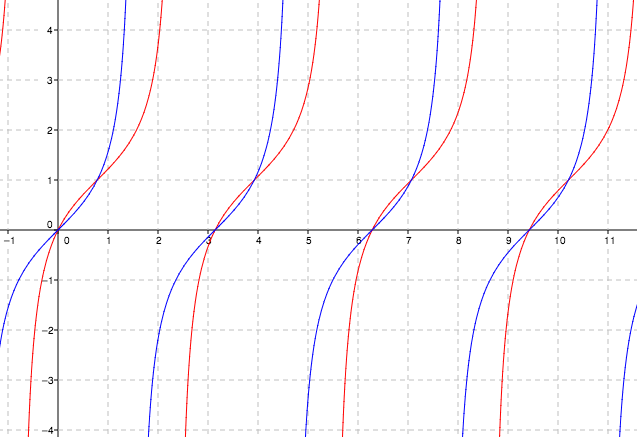Home > CCA2 > Chapter 12 > Lesson 12.1.2 > Problem12-31

12-31.

Graph f(x) = 1 + tan (x). Homework Help ✎Here is the original graph of f(x) = tan(x).How does adding 1 shift the graph?

$\text{How does subtracting }\frac{\pi }{4} \text{ from \textit{x}\, shift the graph?}$